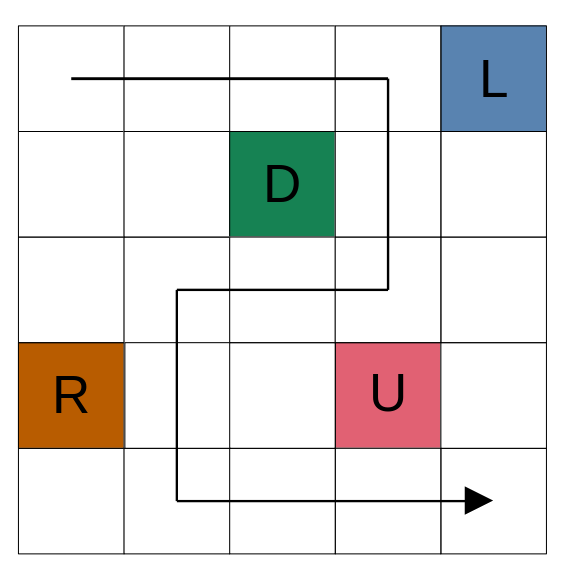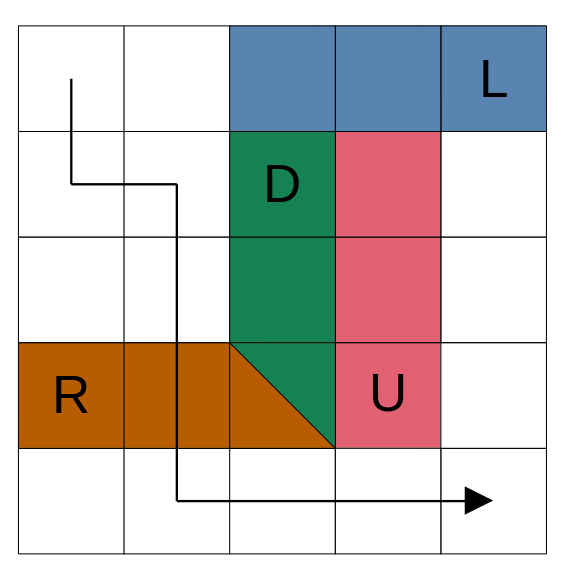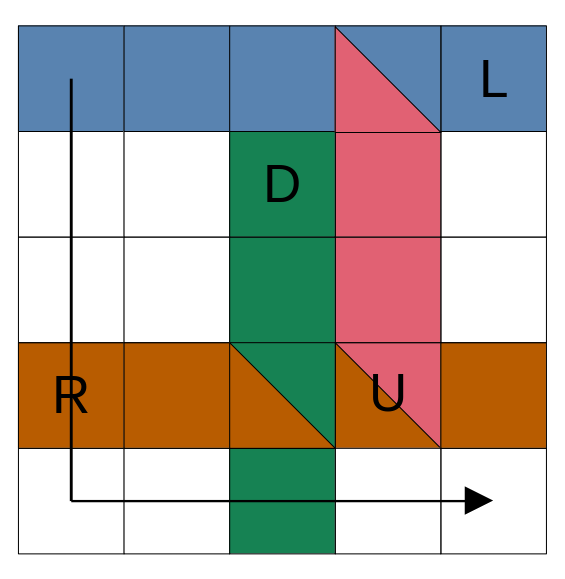November 30, 2023
Latest:
CodeChef Solution

# Snakes on a Grid CodeChef Solution## Problem

There is an �×� grid, with rows numbered 1 to  from top to bottom and columns numbered 1 to  from left to right. The cell at the intersection of row  and column  is denoted (�,�).

Chef is standing at cell (1,1) of the grid, and would like to get to cell (�,�). To do this, he can move one step up/down/left/right from the cell he’s standing at, as long as he remains within the grid.
That is, from cell (�,�) he can move to one of {(�−1,�),(�+1,�),(�,�−1),(�,�+1)}.
Unfortunately, there are snakes in the grid!

Each snake has an initial cell (��,��), and a direction ��. The direction is one of 'U''D''L', or 'R' – denoting up, down, left, and right respectively. This snake acts as follows:

• In the beginning, it occupies only point (��,��).
• At the end of each second, it will extend one unit in its respective direction.
For example, if (��,��)=(4,7) and ��=L, then:

• At first, this snake occupies (4,7)
• After one second, it occupies (4,7) and (4,6) (i.e, it occupies one point more to the left).
• After another second, it occupies (4,7),(4,6), and (4,5); and so on.

Snakes stop extending when they reach the boundary of the grid.
Multiple snakes are allowed to occupy the same cell.
Chef calls a point dangerous if at least one snake occupies it.
The danger of a path from (1,1) to (�,�) is the number of dangerous points it contains.

Help Chef answer  queries of the following form:

• Given , what’s the minimum danger of a path from (1,1) to (�,�) at the start of time ?

### Input Format

• The first line of each test case contains two space-separated integers  and  — the size of the grid and the number of queries.
• The next  lines describe the grid. The -th of them contains a string �� of length , denoting row .
• Each character of the string is one of five from ".LRUD", where '.' denotes an empty cell and the other four denote a snake at that cell with the specified direction.
• The next  lines describe the queries. The -th of them contains a single integer ��, the time for the -th query.

### Output Format

For each test case, output  lines. The -th of them should contain a single integer: the answer to the -th query.

### Constraints

• 1≤�≤300
• 1≤�≤2⋅105
• 1≤��≤106
• �� contains only the characters ".LRUD“.

### Sample 1:

Input

Output

5 3
....L
..D..
.....
R..U.
.....
1
3
6

0
1
3


### Explanation:

The initial grid looks as follows:with one possible path from (1,1) to (5,5) marked out, that has danger 0.
This is the answer to the first query.

The second query has �=3, meaning we’re at the start of the third second. All snakes have moved twice (recall that snakes move at the end of a second). One possible path with danger 1 now is:The third query is �=6, so all snakes have moved five times. One optimal path with danger 3 is:Note that the cells (1,1) and/or (�,�) may themselves be dangerous, in which case they must be counted into the answer as well.